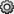## Workspace and Variable Preferences

### Workspace Preferences

Workspace preferences enable you to configure options for saving workspace variables to MATLAB® scripts. They also enable you to restrict the size of arrays on which MATLAB performs calculations, and to specify if you want those calculations to include or ignore `NaN`s.

To open Workspace preferences, on the Home tab, in the Environment section, click. Select MATLAB > Workspace.

PreferenceUsage
Threshold for saving variables to MATLAB script

Specify Maximum array size to limit the number of elements of arrays saved to a MATLAB script.

Specify Maximum struct/object nesting levels to limit the nesting level of structures, arrays, or objects saved to a MATLAB script.

Multidimensional array formatting

Specify how multidimensional arrays are formatted when saved to a MATLAB script.

To create a compact script, select Row vector with reshape (compactness and efficiency).

To retain array representation, select As 2-D pages (readability). Specify the dimensions of a 2-D slice as positive integers less than or equal to the dimensions of the `n`-D array. The second integer must be greater than the first.

File formattingSet the character width at which text in the MATLAB script is wrapped by specifying Maximum characters per line.
Statistical calculations

Set the maximum array size to display statistics for in the Workspace browser using the element and smaller arrays show statistics option. Excluding large arrays from statistical calculations improves Workspace browser performance.

Specify whether `NaN` values are included or excluded from calculations for the statistics displayed in the Workspace browser.

Select Use NaNs when calculating statistics to include `NaN` values or Ignore NaNs when calculating statistics to exclude `NaN` values.

MATLAB array size limit

By default, MATLAB can use up to 100% of the size of RAM (not including virtual memory) of your computer to allocate memory for each MATLAB array. To change this limit to a smaller percentage, select the Limit the maximum array size to a percentage of RAM check box. Then move the slider to adjust the percentage of RAM.

To allow MATLAB to use both RAM and virtual memory when creating an array, clear the Limit the maximum array size to a percentage of RAM check box. If MATLAB attempts to allocate memory that exceeds the resources available on the computer, your system might become nonresponsive.

This limit applies to the size of each array, not the total size of all MATLAB arrays.

### Variables Preferences

When working in the Variables editor, Variables preferences enable you to specify array formatting, cursor movement, and the decimal separator for exporting data using the system clipboard.

To open Variables preferences, on the Home tab, in the Environment section, click. Select MATLAB > Variables.

PreferenceUsage
Format

Select an option from the Default array format to specify the default array output format of numeric values displayed in the Variables editor. This format preference affects only how numbers display, not how MATLAB computes or saves them. For information on formatting options, see the reference page for the `format` function.

Editing

Specify where the cursor moves to after you type an element and press Enter.

To keep the cursor in the element where you typed, clear the Move selection after Enter check box.

To move the cursor to another element, select the Move selection after Enter check box. In the Direction field, specify how you want the cursor to move.

International number handling

In the Decimal separator for exporting numeric data via system clipboard field, specify the decimal separator for numbers you cut or copy from the Variables editor and then paste into text files or other applications.

This preference has no effect on numeric data copied from and pasted into MATLAB. Within MATLAB, decimal separators are always periods.

Some Variables preferences are not available in MATLAB Online™. To show generated code in the Command Window when performing operations in the MATLAB Online Variables Editor, select Show MATLAB code for operations.